### The kinetic theory of gases

An ideal gas does not actually exist but most gases at pressures no more than a few atmospheres come close to this description. The equations that follow describe most situations fairly closely.

The equations can be derived from the kinetic theory of gases, as shown below, and relies on several assumptions most of which are reasonable. (A knowledge and understanding of the assumptions made about an ideal gas is often required in examination). These are that:

1. The gas consists of a large number of molecules.
2. The molecules are in random motion and obey Newton's laws of motion
3. The volume of the molecules is negligibly small compared to the total volume occupied by the gas
4. No forces act on the molecules except during collisions
5. The collisions are elastic (no loss of kinetic energy) and are of negligible duration.

There is a video explanation at the bottom of this page and a PDF copy of these notes here:Kinetic theory notes

but the proof goes like this:

Imagine a single molecule in a box rebounding from one end to another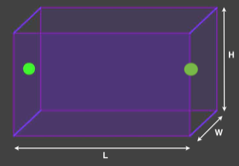To calculate the average force on that face we need the rate of change of momentum.

As it rebounds its momentum changes from one direction to the opposite one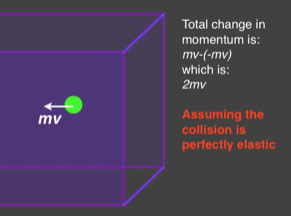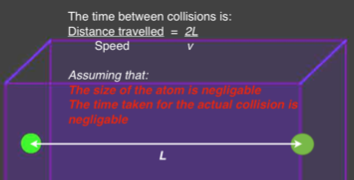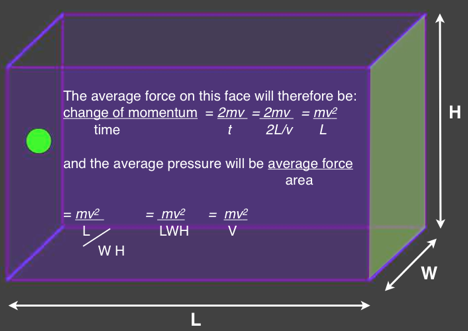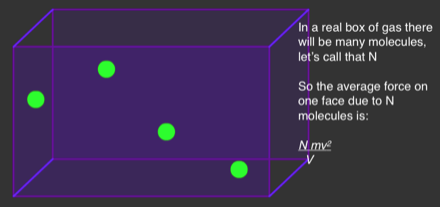but the molecules to not just travel right to left but in all three dimensions and we assume that there are so many molecules and that there motion is random that the vector sum of there velocities in any one direction is the same as in any other.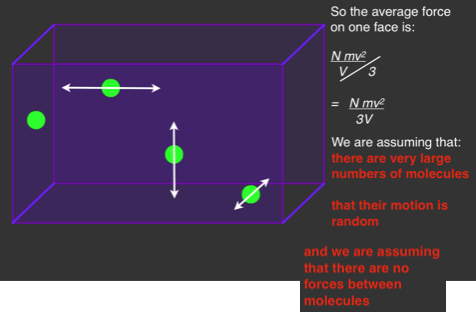The Root Mean Square velocity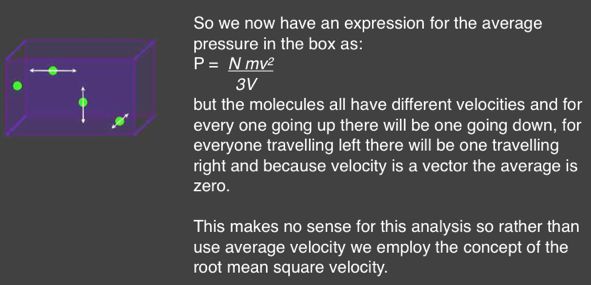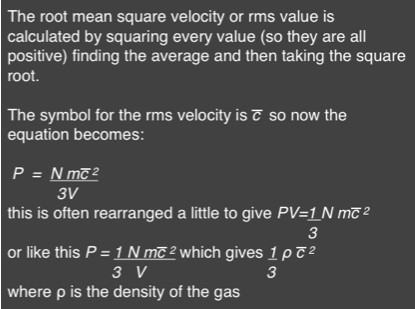### video tutorial – the kinetic theory of gases

Other useful pages on the kinetic theory of gases and the gas laws are: